# Inflation

Once upon a time, tsar owned a money printer and printed and printed. The result of printing money prices went up,in the first year 3.9 %, in the second 6%, in the third 4.7% and in the fourth 5.5%.

Then tsar was failed in election.

Calculate the average annual inflation rate (percentage of price increase) during this four years.

i =  5 %

### Step-by-step explanation:

$i=100\left(\sqrt{\left(1+\frac{3.9}{100}\right)\left(1+\frac{6}{100}\right)\left(1+\frac{4.7}{100}\right)\left(1+\frac{5.5}{100}\right)}-1\right)=5\mathrm{%}$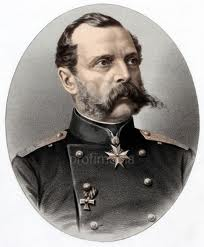Did you find an error or inaccuracy? Feel free to write us. Thank you!Tips to related online calculators
Looking for a statistical calculator?

## Related math problems and questions:

• 3y inflation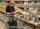Price of the roll rise in the first year by 9%, the second year fell by 5% and in the third year increased by 3%. Calculate the average annual increase in price of the roll.
• Price inflationThis year the average wage grew by 4.5%. Prices rose by 2%. How much did the real wage increase in the given year?
• Annual growthThe population has grown from 25,000 to 33,600 in 10 years. Calculate what was the average annual population growth in%?
• Profit growthThe profit of a company increased by 25% during the year 1992, increased by 40% during the year 1993, decreased by 20% in 1994 and increased by 10% during the year 1995. Find the average growth in the profit level over the four years periods?
• Profitable bank deposit 2012Calculate the value of what money lose creditor with a deposit € 9500 for 4 years if the entire duration is interest 2.6% p.a. and tax on interest is 19%. Annual inflation is 3.7% (Calculate what you will lose if you leave money lying idle at negative int
• Annual increaseThe number of cars produced increased from 45,000 to 47,000 in 3 years. Calculate the average annual increase in cars in%.
• Computer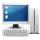The computer was purchased 10000,-. Each year, the price of a computer depreciates always the same percentage of the previous year. After four years, the value of the computer is reduced to 1300,- How many percent was depreciated price of the computer eac
• Volume of woodEvery year, at the same time, an increase in the volume of wood in the forest is measured. The increase is regularly p% compared to the previous year. If in 10 years the volume of wood has increased by 10%, what is the number p?
• 3 years savingsSimple interest problem. Tereza has deposited CZK 100,000 in the bank with an annual interest rate of 1.5%. Interest is added each year and left aside. How many CZK (crowns) will have total after 3 years?
• Enterprise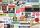The value of annual production enterprise is 7.1 million USD. The average annual growth rate is 1.5%. What will be value of production at the end of the fifth year?
• Percentage increaseWhat is the annual percentage increase in the city when the population has tripled in 20 years?
• Common ratio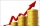If 200 units of a commodity are consumed in a first year, and if the annual rate of increase in consumption is 5% (a) what amount is consumed in the 8th year; (b) in the first 15 years?
• 3 years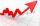The company has increased the number of employees. In year 2005 compared to 2004 13% more, in 2006 18% more against the previous year, in year 2007 20% more compared to 2006. How many % increase in the number of employees in 3 years?
• SavingsThe depositor regularly wants to invest the same amount of money in the financial institution at the beginning of the year and wants to save 10,000 euros at the end of the tenth year. What amount should he deposit if the annual interest rate for the annua
• Precious metals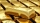In 2006-2009, the value of precious metals changed rapidly. The data in the following table represent the total rate of return (in percentage) for platinum, gold, and silver from 2006 through 2009: Year Platinum Gold Silver 2009 62.7 25.0 56.8 2008 -41.3
• SaleIf the product twice price cut by 25%, what percentage was price cut in total?
• Annual pensionCalculate the amount of money generating an annual pension of EUR 1000, payable at the end of the year and for a period of 10 years, shall be inserted into the bank to account with an annual interest rate of 2%